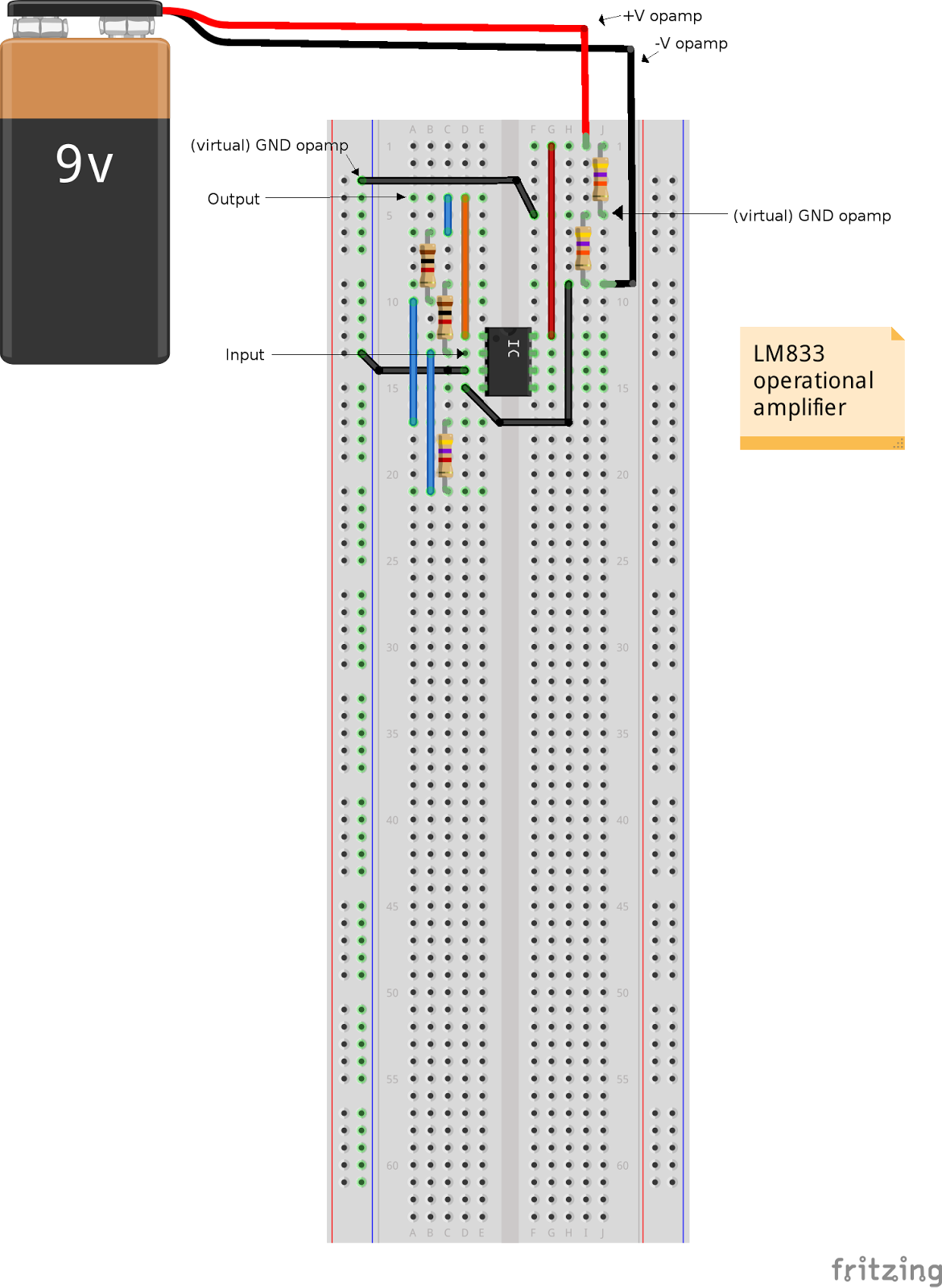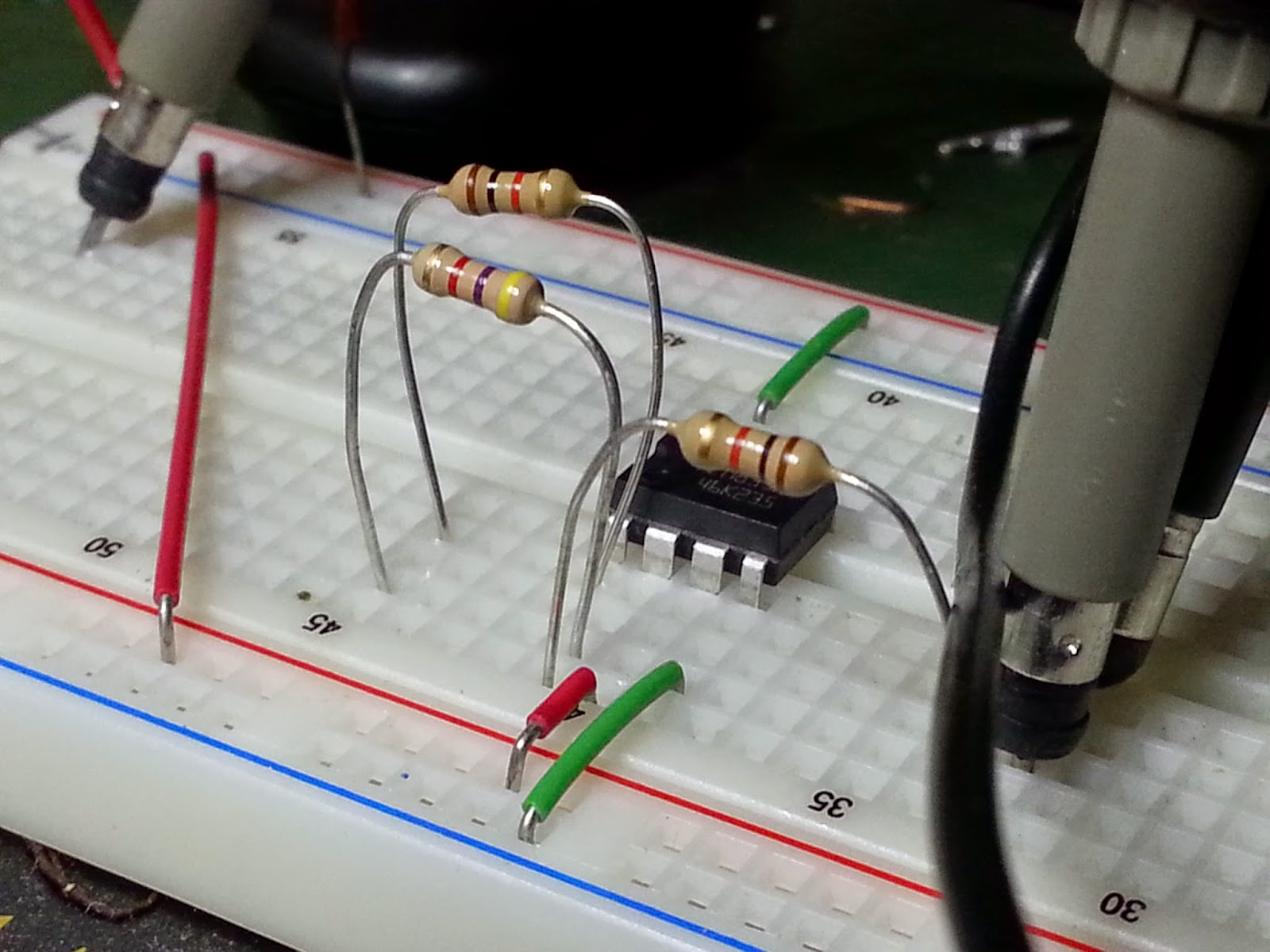## sabato 7 marzo 2015

### How to control an inverting amplifier using Arduino and a digital potentiometer.

An inverting amplifier is a differential amplifier in which that circuit's non-inverting input is grounded, and inverting input is identified with Vinput.An inverting amplifier

The closed-loop gain is R2 / R1, hence Voutput = - R2/R1 Vinput so Voutput/Vinput = G = -R2/R1 and GdB = 20 log(|-R2/R1|) = 20 log(R2/R1). For example, if R1 and R2 where 1kohm and 10kohm, G = 10 V/V and GdB = 20 dB, if R1 and R2 where 2kohm and 15kohm, G = 7.5 V/V and GdB = 17.5 dB. (Inverting amplifier).

If you want to simulate a circuit, you can use the well-known software LTspice. This is what we will do with our amplifier.
First we draw the schematic and then we perform PSPICE schematics circuit simulation (in the time domain and in the frequency domain).An inverting amplifier under test on LTSpice

We want to determine the gain of the amplifier depending on the value of R2 resistor.
In this schematic there is a SPICE directive (.step param R 1k 10k 1k) which determines what resistance to start at (R2 = R), what resistance to stop at (10kohm) , and how much will it increment (1kohm). If we simulate (in the frequency domain) we can see that the GdB value changes according to the R2 value:

R1 = 1KOhm, R2 = 1KOhm, |G| = 1, GdB = 0 dB, Vin-peak = 0.1 V, Vout-peak = 0.1 V
R1 = 1KOhm, R2 = 2KOhm, |G| = 2, GdB = 6 dB, Vin-peak = 0.1 V, Vout-peak = 0.2 V
R1 = 1KOhm, R2 = 3KOhm, |G| = 3, GdB = 9.54 dB, Vin-peak = 0.1 V, Vout-peak = 0.3 V
R1 = 1KOhm, R2 = 4KOhm, |G| = 4, GdB = 12 dB, Vin-peak = 0.1 V, Vout-peak = 0.4 V
R1 = 1KOhm, R2 = 5KOhm, |G| = 5, GdB = 14 dB, Vin-peak = 0.1 V, Vout-peak = 0.5 V
R1 = 1KOhm, R2 = 6KOhm, |G| = 6, GdB = 15.6 dB, Vin-peak = 0.1 V, Vout-peak = 0.6 V
R1 = 1KOhm, R2 = 7KOhm, |G| = 7, GdB = 16.9 dB, Vin-peak = 0.1 V, Vout-peak = 0.7 V
R1 = 1KOhm, R2 = 8KOhm, |G| = 8, GdB = 18 dB, Vin-peak = 0.1 V, Vout-peak = 0.8 V
R1 = 1KOhm, R2 = 9KOhm, |G| = 9, GdB = 19.1 dB, Vin-peak = 0.1 V, Vout-peak = 0.9 V
R1 = 1KOhm, R2 = 10KOhm, |G| = 10, GdB = 20 dB, Vin-peak = 0.1 V, Vout-peak = 1 VSome bode diagrams show the gain changing according to the R2 value

In the picture below you can see the variations of Voutput according to the G value (depending on R2 value)The amplitude of the output signal changes according to the G value

Now we can make a simple prototype with fixed gain. If we choose R1 and R2 equal to 1 kOhm, Rd equal to 4.7 kOhm the gain is G = - (R2 + Rd)/R1 = 5.7 V/V (15.12 dB).Inverting amplifier with fixed gainThe wiring scheme of the inverting amplifier with fixed gainThe inverting amplifier under test

The picture below shows the input (with peak value of 0.42V) and the output signals (with peak value of 2.32V), so the absolute value of the gain is 2.32V/0.42V = 5.52 V/V. You can see from the picture that they are exact opposites (180 degrees out of phase) because this is an inverting amplifier.The values of the input and output signals

Now it's  time to put a digital potentiometer instead of the Rd resistor. For further informations about the digital potentiometer controlled by Arduino, have a look to the article "How to control a digital potentiometer using Arduino UNO"

Connect your circuit as shown in the picture below:The inverting amplifier with the digital potentiometer controlled by Arduino UNO

Download the sketch from our github repository. For the first test we set a Rd value equal to 4.7 kohm so in the sketch the "loop" function should be like that:

void loop() {
int i = 128;                                 // R = 4700 ohm
spi_out(CS_signal, cmd_byte2, i);
}

Load the sketch on Arduino, put a sinusoidal signal in input (amplitude 0.4 - 0.5 V, f = 1 khz) and measure the output.
In my test circuit I have this situation: input signal max peak 0.4V and f = 1 khz, output max peak 2.24V, so the gain is 5.6The values of the input and output signals (digital potentiometer)

Now we change dinamically the gain of the amplifier, switching between two values of Rd (4.7 kohm and 9 khom) so in the sketch the "loop" function should be like that:

void loop() {
int i = 128;                                                                // R = 4700 ohm
spi_out(CS_signal, cmd_byte2, i);
Serial.print("value i = ");
Serial.print(i);
Serial.println("   R = 4700 ohm");
delay(5000);

i = 0;                                                                      // R = 9000 ohm
spi_out(CS_signal, cmd_byte2, i);
Serial.print("value i = ");
Serial.print(i);
Serial.println("     R = 9000 ohm");
delay(5000);
}

In the clip below you can see the result of this test:

The gain switches between 5.4 (corresponding to a Rd = 4.7 kohm) and 9 (corresponding to a Rd = 9 kohm).

In the video below I show the value of Rd measured using a digital multimeter (without the op amp)